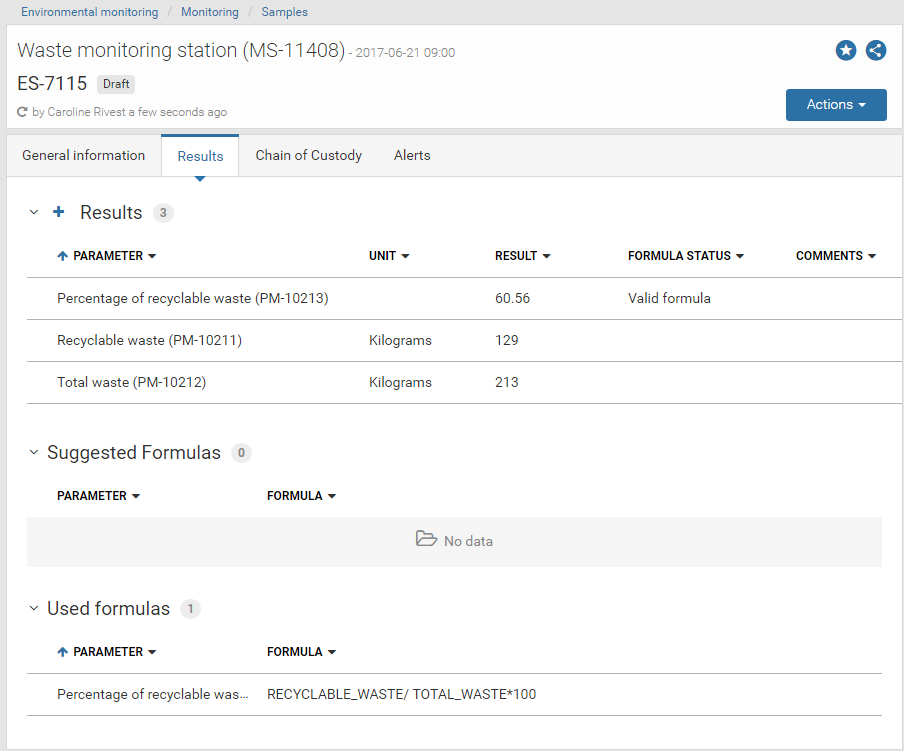# Calculate Complex Parameters

How to create calculated parameters
Parameters are managed in the Parameters register. When adding a new parameter, select Calculated value as the Input format. Once this format is selected, four additional fields become available which allow you to customize your formula.

For example, you might want to know the Percentage of recyclable waste. However, the two parameters for which information is collected are the Total waste (kg) and the Recyclable waste (kg). The example below shows how to enter the formula to calculate the percentage of recyclable waste.Formula field
The Formula field is used to enter the formula to be calculated for your parameter. Accepted symbols, operators and variables are the followings:

• Mathematical operators: +, -, *, ^, % and /
• Parentheses: '(' and ')'
• Numbers
• Import code of available parameters

Note: Any available parameter can be added to the formula. However, they must have at least one monitoring program in common with the new parameter.

Available parameters field
Available parameters are listed in the Available parameters field. You can select parameters to automatically add them to the formula without having to type their reference code manually.

A parameter is available if:

• its input format is "Numeric" or "Numeric with symbol"
• it has at least one monitoring program in common with the new parameter
• it has a valid reference code (letters, numbers and underscore symbol characters only and a minimum of one letter)

Decimals field
Default decimals number is set to 2. It can be customised to any other number.

If the Add to results automatically box is checked a result will automatically be added for this parameter in all samples & surveys where at least one of the parameters used in the formula is entered.

If the box is left blank the result will not automatically be calculated but will be listed in the suggested formulas section.

How to work with calculated parameters (samples/surveys form)Results section
The Results sections shows both, results entered for parameters and results for the calculated paremeters. If the Add to results automatically box has been ckecked when creating the calculated parameter, this parameter is automatically added to the Results section. If not, it has to manually be added (see Suggested formulas section).

Results of calculated values:

• can be removed but not modified
• are updated if results of their parameters or the formula change
• Formula status is marked as
- Incomplete formula if one or more parameters results are missing
- Invalid formula if there is an error (e.g Division by a parameter with zero value)
- Valid formula if the formula and its parameters are valid

Note: if a formula is marked as Invalid, the activation of the sample or the survey will be blocked (Actions blue button -> Activate option)

Suggested formulas section
The Suggested formulas section shows formulas that meet the following criteria:

• are not showed in the Results section
• have at least one valid parameter
• have the same monitoring program as the sample/survey

Used formulas section
The Used formulas section shows calculated parameters that are listed in the Results section.